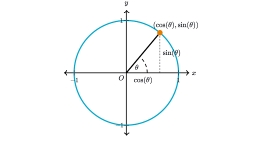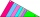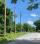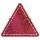# Q-Exam

If tg α = 9.6, Calculating sin α, cos α, cotg α .

cotg α =  0.1
sin α =  0.1
cos α =  0.99

### Step-by-step explanation:Did you find an error or inaccuracy? Feel free to write us. Thank you!Tips to related online calculators
Most natural application of trigonometry and trigonometric functions is a calculation of the triangles. Common and less common calculations of different types of triangles offers our triangle calculator. Word trigonometry comes from Greek and literally means triangle calculation.
Pythagorean theorem is the base for the right triangle calculator.

#### You need to know the following knowledge to solve this word math problem:

We encourage you to watch this tutorial video on this math problem:

## Related math problems and questions:

• CotangentIf the angle α is acute, and cotan α = 1/3. Determine the value of sin α, cos α, tg α.
• Trigonometric functionsIn the right triangle is: tg α= frac(2) 1 Find the value of s and c: sin α= (s)/(√ 5) cos α= (c)/(√ 5)
• TrigonometryIf you know that cos(γ) = sin (806°), what is the angle γ?
• Sin cos tanIn triangle ABC, right-angled at B. Sides/AB/=7cm, /BC/=5cm, /AC/=8.6cm. Find to two decimal places. A. Sine C B. Cosine C C. Tangent C.
• SinesIn ▵ ABC, if sin(α)=0.5 and sin(β)=0.6 calculate sin(γ)
• TrigonometryIs true equality? sin(x +13 π)= sin(x)
• RightDetermine angles of the right triangle with the hypotenuse c and legs a, b, if: 3a +4b = 4.9c
• Right triangle trigonometricsCalculate the size of the remaining sides and angles of a right triangle ABC if it is given: b = 10 cm; c = 20 cm; angle alpha = 60° and the angle beta = 30° (use the Pythagorean theorem and functions sine, cosine, tangent, cotangent)
• Three pillarsOn a straight road, three pillars are 6 m high at the same distance of 10 m. At what angle of view does Vlado see each pillar if it is 30 m from the first and his eyes are at 1.8 m high?
• CosineThe point (3, 4) is on the terminal side of angle θ. cos θ = ...
• Regular n-gonWhich regular polygon have a radius of circumscribed circle r = 10 cm and the radius of inscribed circle p = 9.962 cm?
• Right angled triangle 3Side b = 1.5, hypotenuse angle A = 70 degrees, Angle B = 20 degrees. Find its unknown sides length.
• Tetrahedral pyramidDetermine the surface of a regular tetrahedral pyramid when its volume is V = 120 and the angle of the sidewall with the base plane is α = 42° 30'.
• Regular 5-gonCalculate the area of the regular pentagon with side 7 cm.
• EQL triangleCalculate the inradius and circumradius of an equilateral triangle with side a=77 cm.
• TreeBetween points A and B is 50m. From A we see a tree at an angle 18°. From point B we see the tree in three times bigger angle. How tall is a tree?
• KLM triangleFind the length of the sides of the triangle KLM if m = 5cm height to m = 4.5 cm and size MKL angle is 70 degrees.